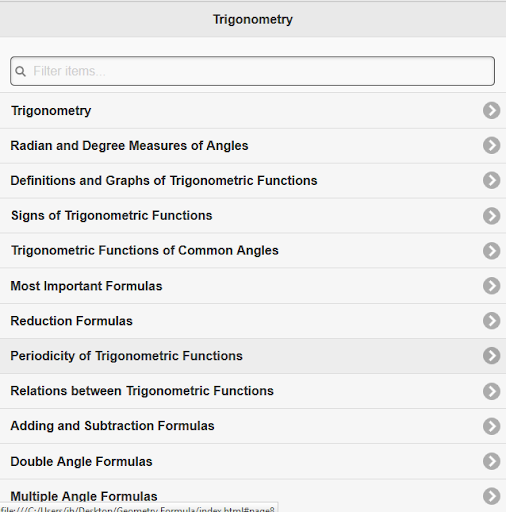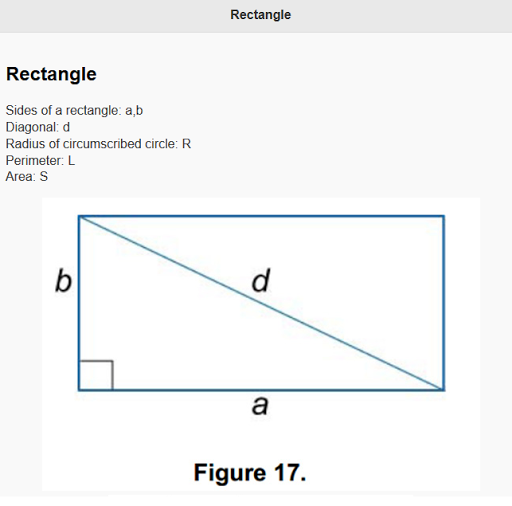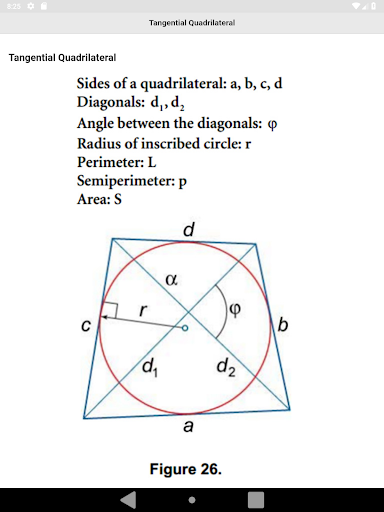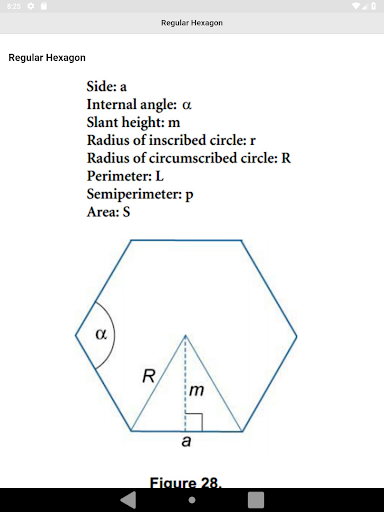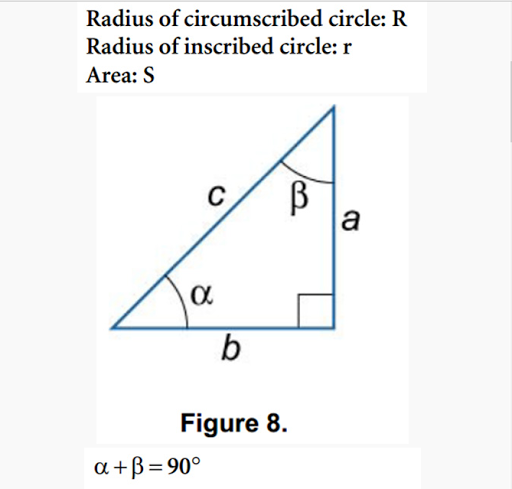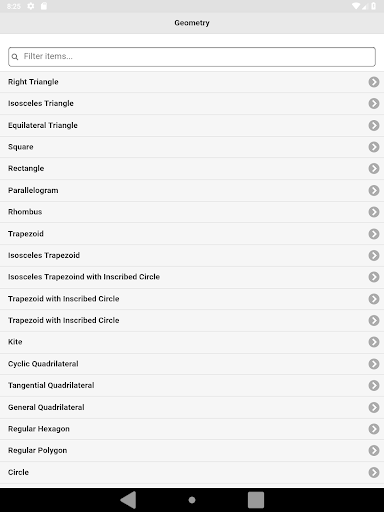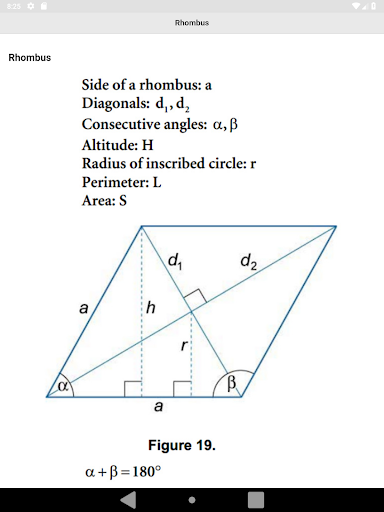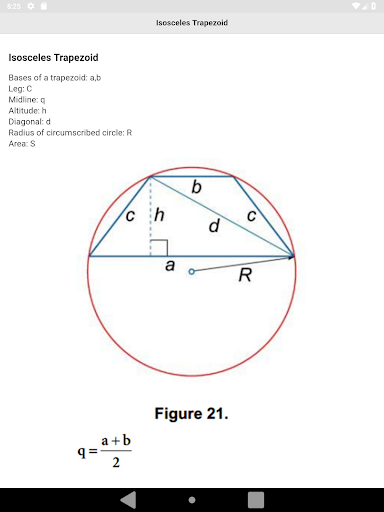All Geometry Formula for all math students. You will able to search Formulas in this app. This app contain these formulas:

# Trigonometry
# Radian and Degree Measures of Angles
# Definitions and Graphs of Trigonometric Functions
# Signs of Trigonometric Functions
# Trigonometric Functions of Common Angles
# Most Important Formulas
# Reduction Formulas
# Periodicity of Trigonometric Functions
# Relations between Trigonometric Functions
# Double Angle Formulas
# Multiple Angle Formulas
# Half Angle Formulas
# Half Angle Tangent identities
# Transforming of Trigonometric Expressions to Product
# Transforming of Trigonometric Expressions to Sum
# Powers of Trigonometric Functions
# Graphs of Inverse Trigonometric Functions
# Inverse Tangent Function
# Inverse Cotangent Function
# Inverse Secant Function
# Inverse Cosecant Function
# Principal Values of Inverse Trigonometric Functions
# Relations between Inverse Trigonometric Functions
# Trigonometric Equations